# Functional Transcendence on Complex Manifolds Uniformized by Bounded Symmetric Domains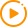Watch Video

11 18, 2020

Speaker: Professor Ngaiming Mok University of Hongkong

Time:  3:30-4:30pm, November 18, 2020.

Venue:N202

TitleFunctional Transcendence on Complex Manifolds Uniformized by Bounded Symmetric Domains

AbstractFinite-volume quotients of bounded symmetric domains ？, which are naturally quasi-projective varieties, are objects of immense interest to Several Complex Variables, Algebraic Geometry, Arithmetic Geometry and Number Theory, and an important topic revolves around functional transcendence in relation to universal covering maps of such varieties (in analogy to the exponential map exp :). While a lot has already been achieved in the case of Shimura varieties (such as the moduli spaceof principally polarized Abelian varieties) by means of methods of Diophantine Geometry, Model Theory, Hodge Theory and Complex Differential Geometry, techniques for the general case of not necessarily arithmetic quotients ？/Γ =:have just begun to be developed. For instance, uniformization problems for subvarieties of products of arbitrary compact Riemann surfaces of genus ≥ 2 have hitherto been intractable by existing methods. An important link between the Arithmetic Geometry and Complex Differential Geometry on Shimura varieties is the result of Moonen (1998) according to which weakly special varieties in the arithmetic context are exactly the totally geodesic subsets with respect to canonical Khler metrics in the differential geometric context. We will explain a differential geometric approach leading to characterization results for totally geodesic subvarieties offor the universal covering map π:？→. Especially, we will explain how uniformization theorems for bi-algebraic varieties can be proven by analytic methods involving the Poincar-Lelong equation in the cocompact case (joint work with S.-T. Chan), generalizing in the cocompact case earlier results of Ullmo-Yafaev (2011) in the case of arithmetic quotients. More generally, we will consider the Zariski closures of images of algebraic sets under the universal covering map π:？→. In the arithmetic case, Klingler-Ullmo-Yafaev (2016) resolved the hyperbolic Ax-Lindemann Conjecture (which is one of the two major components for the confirmation of the Andr-Oort Conjecture for Shimura varieties) in the affirmative ascertaining that such Zariski closures are weakly special (equivalently totally geodesic). I will explain how the arithmeticity condition can be dropped in the rank-1 case by a completely different proof using foliation theory, Chow schemes and Khler geometry.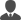Contacts: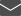E-mail: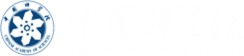•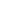Facebook
•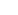Twitter
•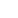Instagram
•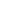Snapchat
•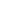LinkedIn
•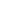YouTube
•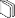Social Media Directory